## Sample Test Problems

1. A hydrogen atom is in the,state. To which states is it allowed to decay via electric dipole transitions? What will be the polarization for a photon emitted along the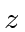-axis ifdecreases by one unit in the decay?

2. Derive the selection rules for radiative transitions between hydrogen atom states in the electric dipole approximation. These are rules for the change in, and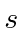.

3. State the selection rules for radiative transitions between hydrogen atom states in the electric dipole approximation. These are rules for the allowed changes in,, and parity. They can be easily derived from the matrix element given on the front of the test. Draw an energy level diagram (up to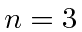) for hydrogen atoms in a weakfield. Show the allowed E1 transitions fromtoon that diagram.

4. Calculate the differential cross section,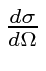, for high energy scattering of particles of momentum p, from a spherical shell delta function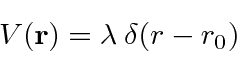Assume that the potential is weak so that perturbation theory can be used. Be sure to write your answer in terms of the scattering angles.
5. Assume that a heavy nucleus attractsmesons with a weak Yakawa potential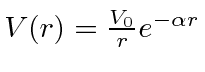. Calculate the differential cross section,, for scattering high energymesons (mass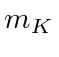) from that nucleus. Give your answer in terms of the scattering angle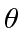.

Jim Branson 2013-04-22